#Original Article
• Topology Optimization of a Lightweight Multi-material Cowl Cross Member Using Matrix Input with the Craig Bampton Nodal Method
• Dong il Son*, Sangwoo So**, Dong hyuk Choi*, Daeil Kim***†

• * R&D Center, Dongkook Ind. Co., Ulsan
** Automotive Parts Institute & Center, Ulsan Technopark

Abstract

As demand of light weight in the automotive industry has increased, the cowl cross member has been investigated using various methods to change the material. Conventionally, a cowl cross member has been made of steel and aluminum, but recently researchers tested multi-material such as aluminum and plastic. We studied a new model of the cowl cross member made of composite and non ferrous materials. For products with a high degree of freedom in design, generally, the method of topology optimization is advantageous and for the partial bracket part of the cowl cross member had a degree of freedom in the design, a topology optimization is appropriate. Considering the characteristics of the cowl cross members, we need research to minimize the weight while having the performance of noise, vibration and harshness(NVH). Taking the mounting status of the product into consideration, we used an assembly model to optimize the cowl cross member. But this method took too much time so we considered simple cowl cross member assemble conditions using the direct matrix input method(DMI) with the Craig-Bampton Nodal Method. This method is capable of considering the status of the assembly without assembling the model, which reduced the solving time and increased the accuracy comparison with a cowl cross member without DMI.

Keywords: : DMI (Direct matrix input), Craig-Bampton nodal method, Topology optimization, Super-element, NVH (Noise, vibration and harshness), Multi-material

introduction

Recently, the automotive industry has been going toward xEV (BEV, HEV, PHEV, FCEV) and smartification so the curb weight has been increased by additional parts such as batteries, motors, and electric parts. Light weight is a very important factor in developing automotive parts in the automotive industry. A cowl cross member is made of many parts, and each serves a special purpose in the structure . The cowl cross member(CCM) supports the instrument panel (IP) system and the relevant NVH performance. For several decades, many car makers have been developing various CCMs to achieve light weight and satisfactory NVH performance. For sake of light weight, the material of the CCM has been changed from steel to aluminum, magnesium and a hybrid material made of steel and engineering plastics. In this study, we propose a hybrid material CCM made of magnesium, aluminum, and polyamide6-glass fiber to achieve light weight and NVH performance.
In particular, nylon6-glass fiber composite materials have relatively strong thermal and shock characteristics among polymeric materials, which are applicable to automotive parts that are currently undergoing changes to plastic materials, which are increasing [2,3].
The CCM is a main component of the car body frame and contributes to the overall integrity and stiffness that affects the frequency response of the entire vehicle. The CCM is the part that transfers the load from the steering column to the body through its junctions with the car body on the sides, top and bottom. Both ends of the CCM meet the A-Pillar on the side, and the middle end point meets the Center floor . The brackets of the fixed points to the A-Pillar and the Center floor can be made of composite materials and magnesium materials that does not consider the direction of flow, so it can have a high degree of freedom in design due to manufacturing the injection molding for engineering plastics and die casting for magnesium. In this case, the design of the bracket is suitable for topology optimization because topology optimization has been primarily applied for the preliminary design of solid structures, and mechanical parts had a high degree of freedom in the design .
In terms of the role of the CCM, we have to consider the performance of NVH transmitted from the automotive body, so the CCM must consider the assembly conditions. In the topology optimized process, much time was spent to solve the assembly model, the so-called full model. For this reason, we investigated the DMI method with the Craig-Bampton Nodal Method which could consider the assembly conditions without an assembly model. In this case, we defined the super-element of the CCM which meets the A-Pillar on the side and the center floor on the middle. Using the super-element, we accepted the effect of the assembly conditions to use only the CCM component. The super-element defines as an external node connected rigid body that facilitates sharing models between the design group and the non-design group [6,7] and expresses the performance of NVH transmitted by the non-design group through the reduced matrices that accomplishes a topology optimization.
There are two approaches in a topology optimization method: the density method  and homogenization methods .
In this paper, first, we suggest a reduced model of CCM assembly with the Craig-Bampton procedure based on free interface modes and where the substructures are assembled through the interface force. Second, we suggest the optimized CCM model using the topology optimization method with the density method.

theoretical analysis

2.1 Craig-Bampton nodal method
The Craig-Bampton method is especially well suited for a sub-structuring problem . In the course of a finite element solution, the matrix representation of a structure’s stiffness, mass, damping, and loading are generated. The system equations using these matrices are solved to simulate the structures’ behavior. The time taken for these matrix solutions is generally proportional to the square of the number of degrees of freedom of the structure . To save simulation time, the non-design portion of the structure is removed and the design portion of the structure is retained using the super-element by the method of reduced matrices.
The Craig-Bampton reduction basis consists of a combination of static condensation modes and eigenmode of the fixed, undamped structure .
The following is common to all super-element generation methods and the details of the initial partitioning step involved in generating the reduced matrices.
The linear static equations can be expressed by: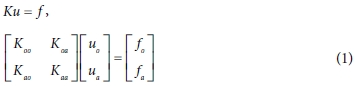Here, the subscript o denotes the inner degree of freedom, and the a denotes the degree of freedom of the interface.
K is the stiffness matrix and u and f are the displacement and force vectors.
The two resulting equations yield: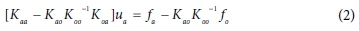This equation can be expressed as follows: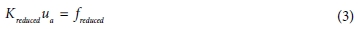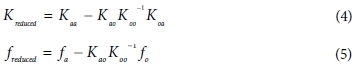A coordinate transformation u = Tuo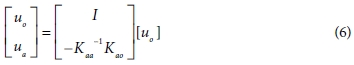The reduced stiffness matrix can be written by: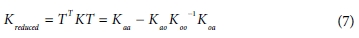Then with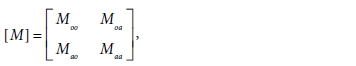the reduced mass matrix becomes: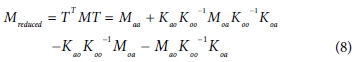An eigenvalue analysis is performed on the reduced matrices as follows: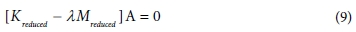where λ is an eigenvalue and A is the partitioned eigenvectors of the system.
A normal modes analysis of the fixed-interface system yields the diagonal matrix of eigenvalues Dω and the matrix of eigenmodes Aω . The column dimension of Aω can select the cut-off frequency or the number of modes. The static displacement modes are the matrix As and the interface forces fa.

In this field, T is written as T = [Aω As].
The reduced stiffness matrix and mass matrix are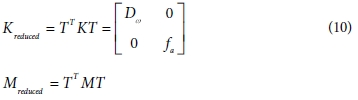2.2 Topology optimization
The object of optimization is to accelerate the design process and to increase the efficiency of the final design, thus providing maximum performance using minimal materials. Thus for the design of a new product, the method of topology optimization is the most effective based on the material distribution method and the calculated optimized size by modifying the stiffness matrix to depend on material density that is allowed to vary continuously between a solid and a void [5,12].
In this study, we used the material distribution to complete the optimization under given constraints, which is gradient-based optimization method.
First, to avoid resonance in a structure and to meet the NVH requirement, it is important that the optimal topology be within the proper range of the structure’s natural frequency . The natural frequencies of the CCM must be different due to different boundary situations generated by attached structures.
In general, when a vehicle is operating, the resonance value can be determined by the engine’s vibration and structure of the power train . Hence, the car makers suggest the value of natural frequency that is over 38 Hz at the first mode.
The optimization problems statement of the CCM is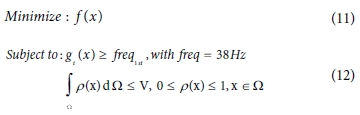where f(x) is the mass function and g(x) is the frequency function and ρ(x) is the density function, Ω is volume and W is a design domain.

simulation

3.1 Topology optimization of CCM with DMI
For the sake of topology optimization of the CCM, we used the direct method input(DMI), which is a reduced model. The process of the simulation is 1) we accomplish the modal analysis of the full model, 2) we generate the DMI model using the reduced model, 3) we optimize the CCM with the super-element considering the boundary of the full model and lastly, we analyze the modal analysis of the full model including the optimized CCM.
In this optimization process, the objective and the constraint functions are as follows;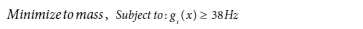The process of simulation is shown in Fig. 1. The analysis of the simulation uses the commercial software OptistructTM. In the case of full model with the CCM concept model, the first mode frequency is 45.1 Hz and the second mode frequency is 47.4 Hz.
As shown in Fig. 2, the super-element is at the location of hard point and the super-element is the part of the CCM that is connected by a rigid body and the B.I.W. corresponding to the target CCM and the boundary condition.
Fig. 3 shows the full model considering the mounting status of the CCM in B.I.W.
The component models to optimize are the mounting bracket, the side mounting bracket, and the center support as expressed by Fig. 4.
The materials of components are magnesium and composite (PA6-GF60: 60% long strand glass fiber reinforced nylon 6 Natural) and the cowl pipe as a non-design part is made of aluminum. Table 1 shows the materials and properties applied to each part as shown Fig. 4.
We use the topology optimized conditions to minimize the mass and to raise the value to 38 Hz; the design is illustrated by Fig. 5. The target components were optimally designed only for parts that significantly affect a NVH performance. The rest of the parts refer to the existing cowl cross member geometry.
In addition, we designed the new model as in Fig. 6 based on the topology optimized model of the CCM to consider manufacturing conditions such as the plastic injection process and magnesium die casting process.
In the NVH performance of the optimized model the first natural frequency is 38.37 Hz and second natural frequency is 39.58 Hz and the weight is 5.148 kg. Based on the first natural frequency value, the result of the NVH performance of both the full model and only the CCM using the super-element are 38.33 Hz and 38.37 Hz, respectively. Fig. 7 shows the primary natural frequency and mode shape with super-element and full model. There is little difference in results. On the other hand, the number of nodes of the CCM model and the full model including the CCM are 62,713 nodes and 790,202 nodes, respectively. The size of model for NVH performance analysis considering BIW mounting conditions has been reduced to 1/12. Moreover, the solving time is 39 times faster on the basis of 4 CPUs of 2.60 GHz. Table 2 shows the size and analysis time of full and the reduced models.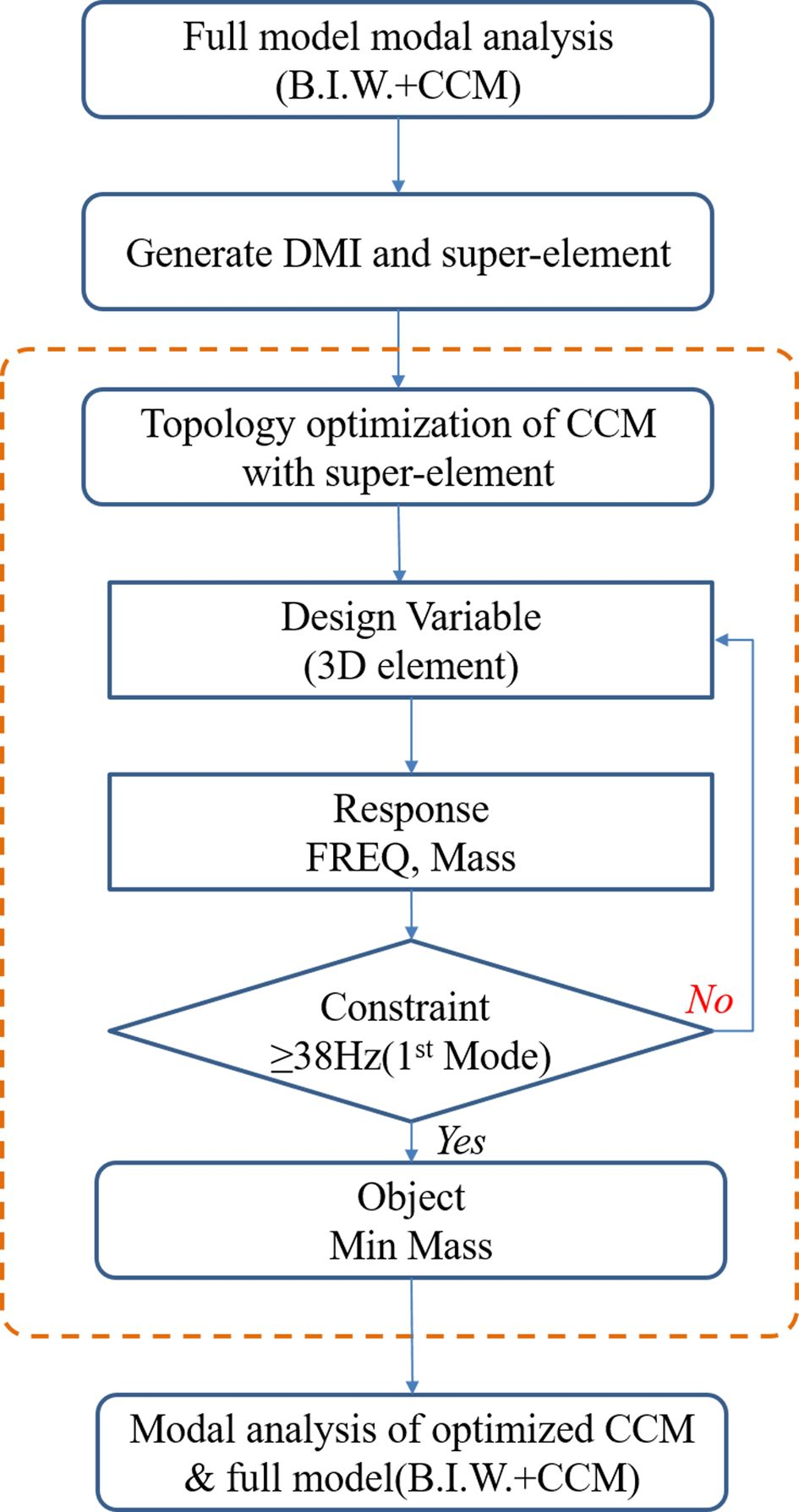Fig. 1 The process of topology optimization of the CCM using DMI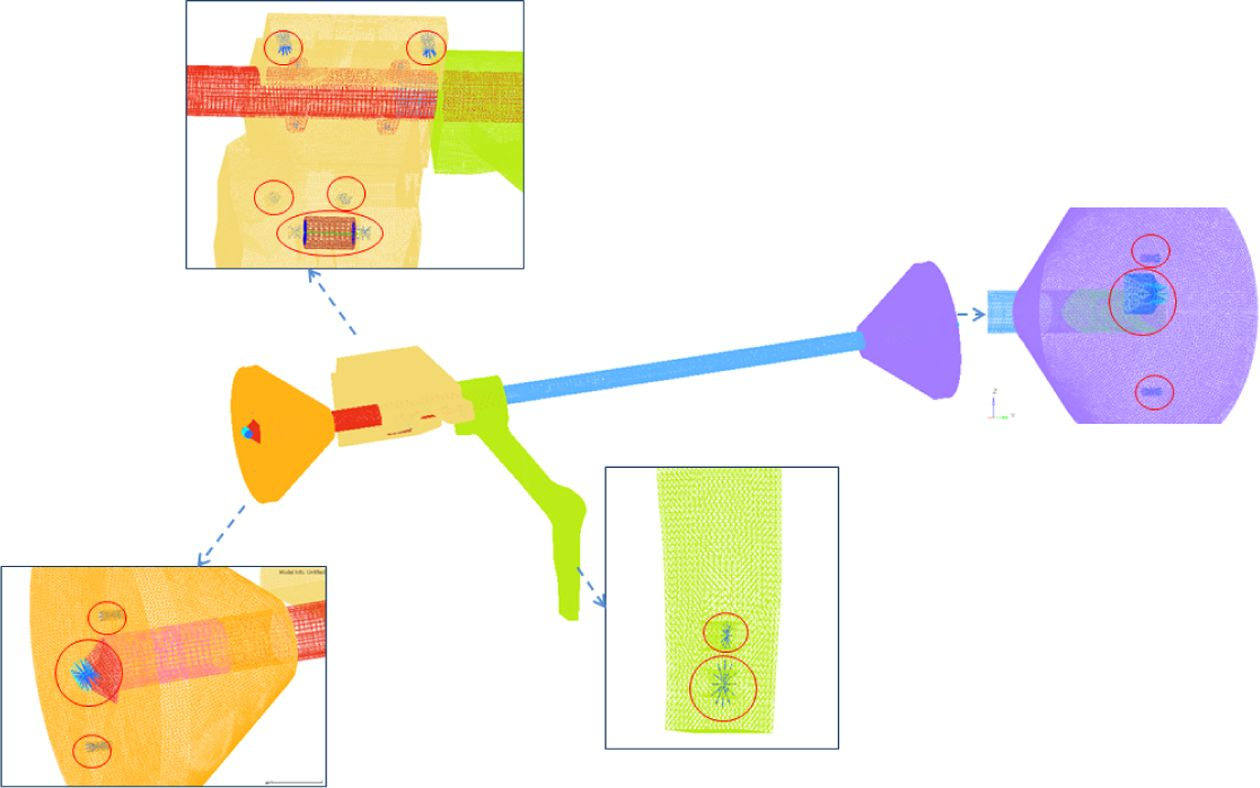Fig. 2 Super-element assignment (The red circle denote superelement)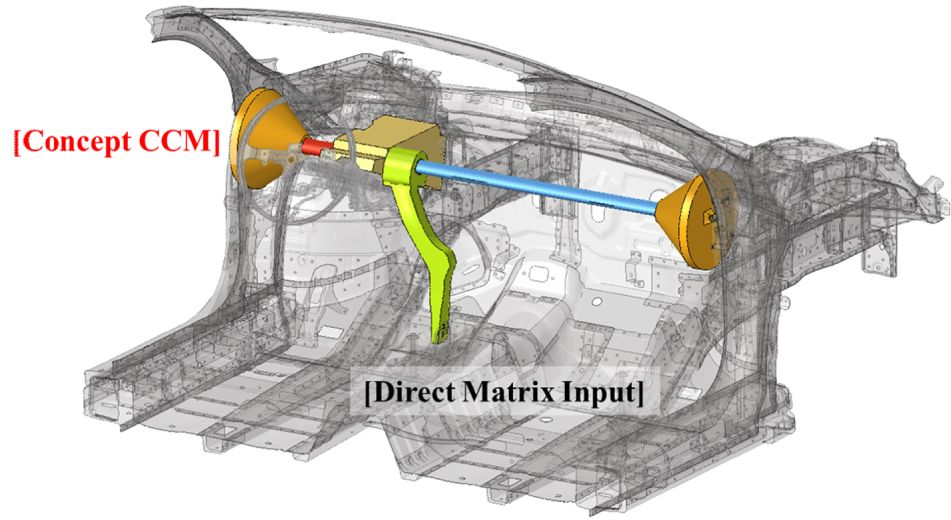Fig. 3 Full model included the concept model of the CCM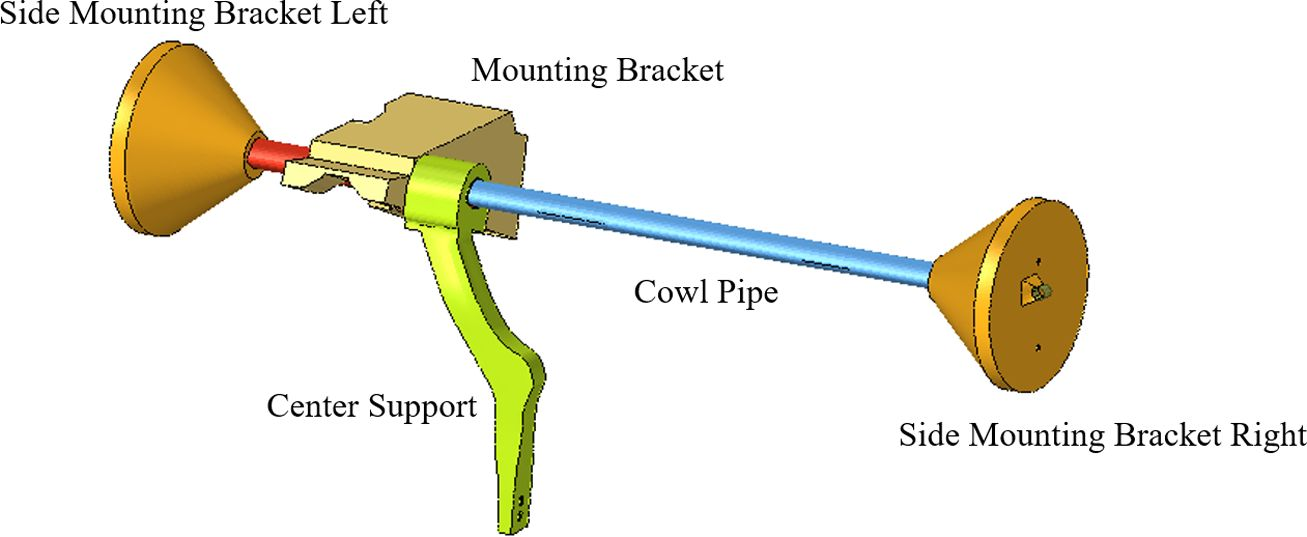Fig. 4 The concept model of the CCM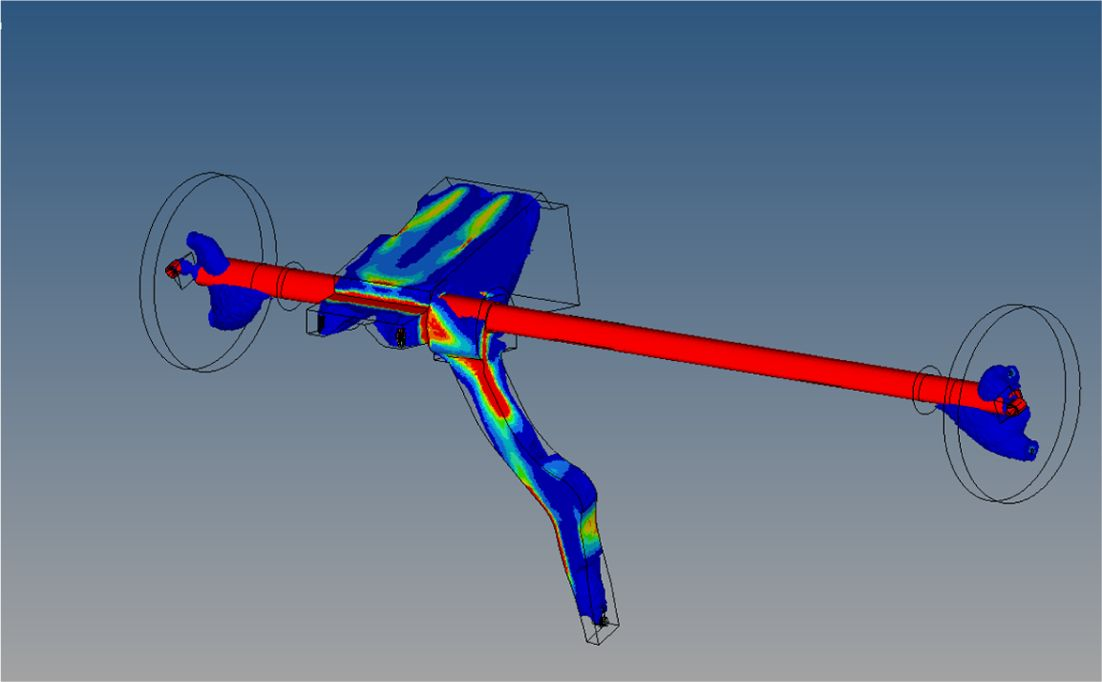Fig. 5 The topology optimized model of the CCM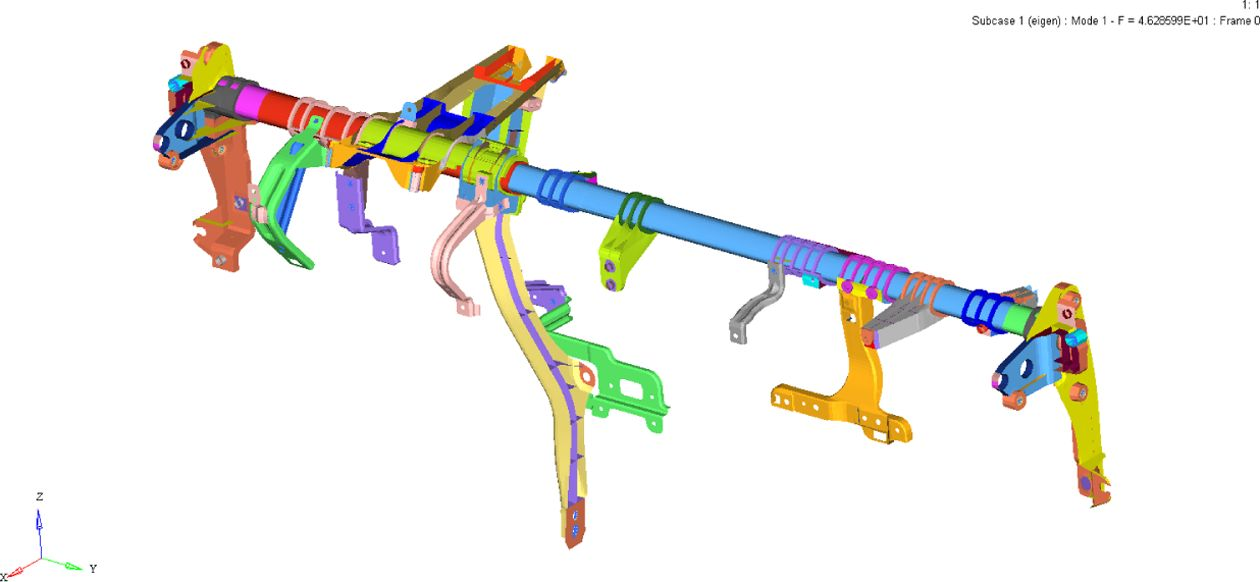Fig. 6 The optimized model of the CCMFig. 7 1st and 2nd natural frequency of the CCM using the superelement and full model
 Table 1 Material parameter of components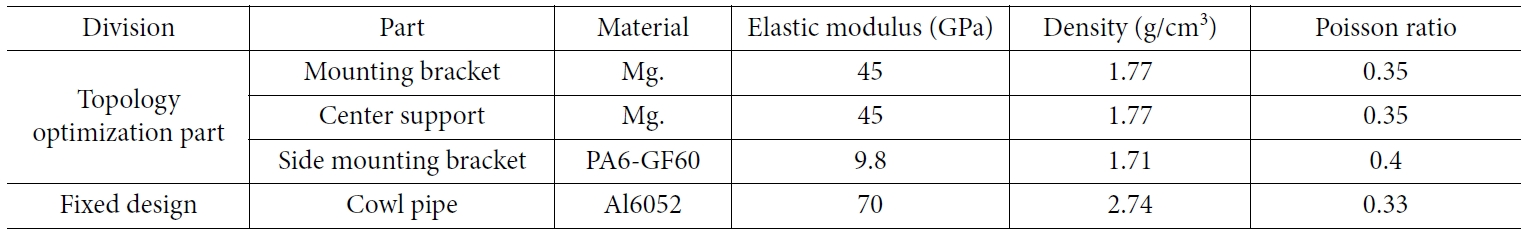Table 2 The size of model & solving time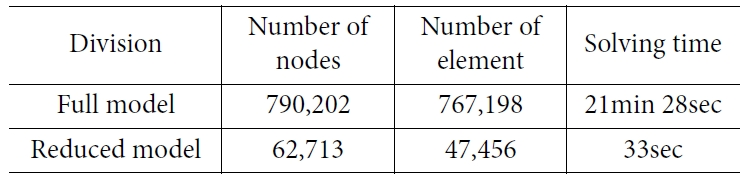experiment

4.1 Modal test of the CCM
Based on the optimized design as shown in Fig. 6, we made the CCM as in Fig. 8 and estimated the natural frequency as in Fig. 9 which is a non-constraint; in other words, the boundary conditions are free-free conditions and measure 6 points. The condition of the vibration input is that the brittle point of the CCM is impacted by the impact hammer (B&K 8206-002) in the vertical direction and uses the 3-axis acceleration sensor (B&K 4506B).
The result of the test was as follows: the first natural frequency is 40.8 Hz and the second natural frequency is 43.7 Hz as shown Fig. 10 and Fig. 11.
Table 3 summarizes the results of the simulation and experiments. In the result of experiment and simulation, the difference of the frequency value was less than 2.5 Hz and the percentage was about 6%. Although we expected that an error might occur from the experiment and manufacturing, the result of the simulation can be considered as reliable. Comparing other CCMs made of steel material of a similar vehicle model with the hybrid material CCM in this study, the weight of the steel type and hybrid type are 8.7 kg and 4.79 kg, respectively.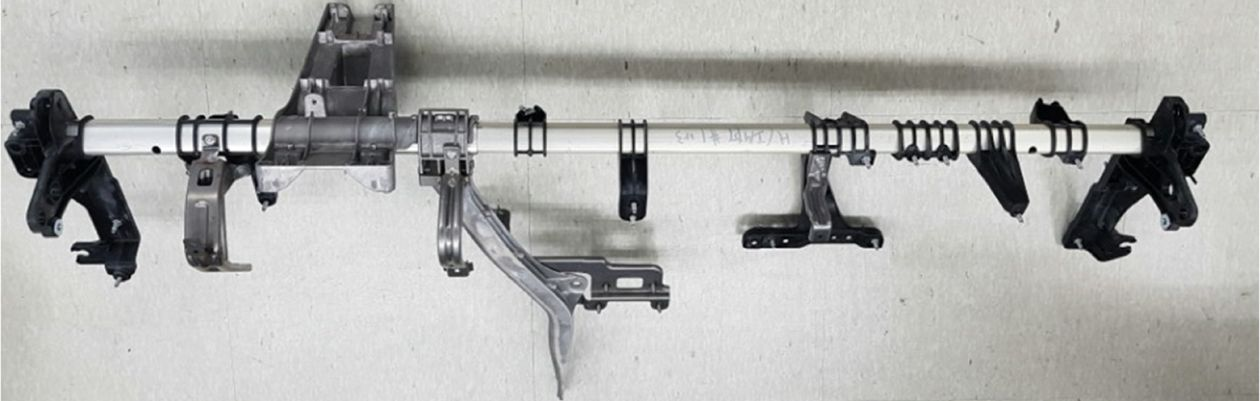Fig. 8 The product of the CCM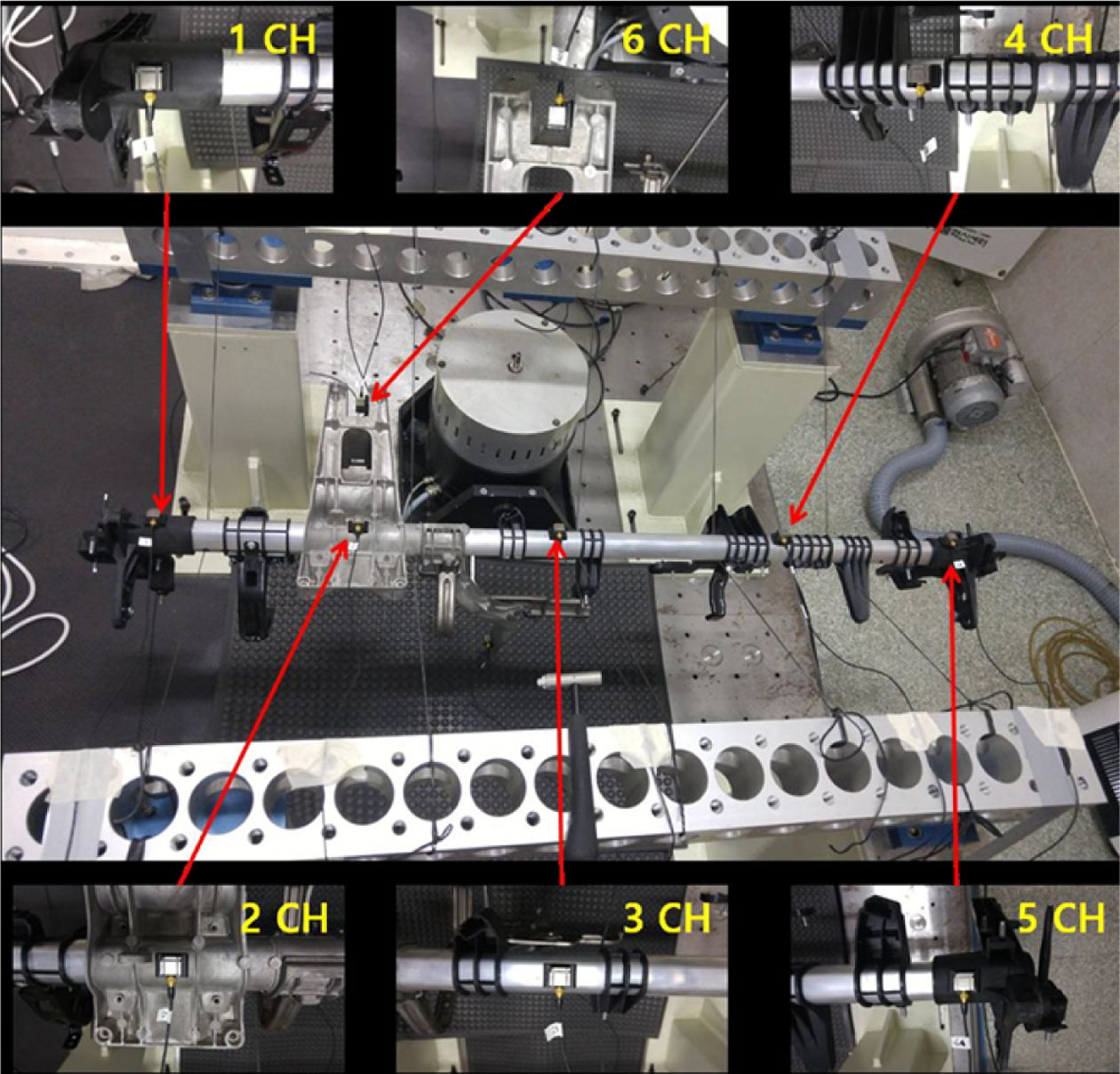Fig. 9 Experimental set up of the CCM for estimating natural frequency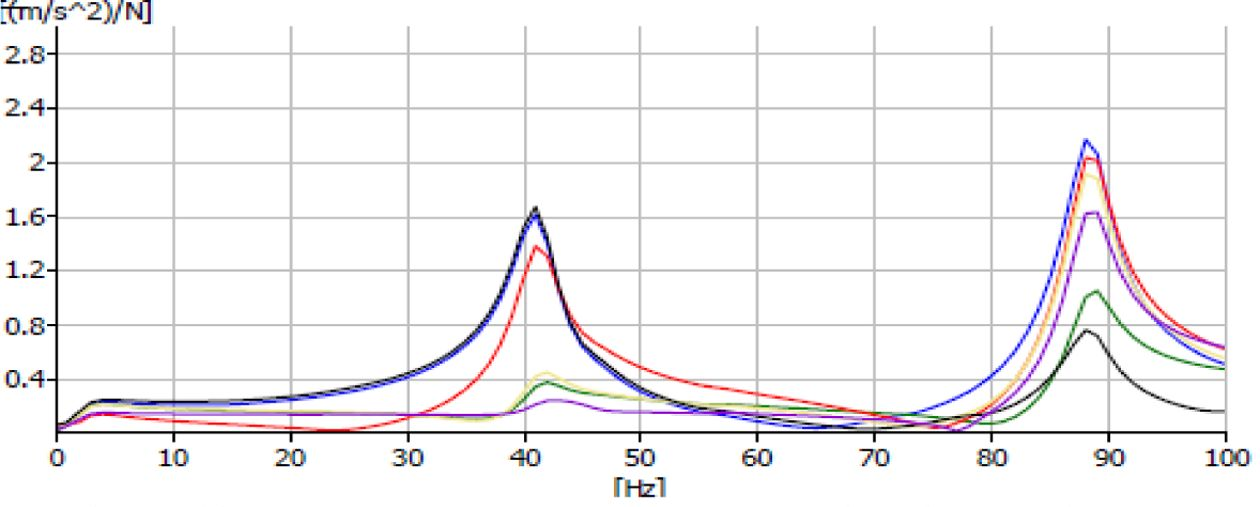Fig. 10 Frequency response graph at Z direction(vertical) –40.8 HzFig. 11 Frequency response graph at X direction (longitudinal)– 43.7 Hz
 Table 3 The result of frequency of the test and simulation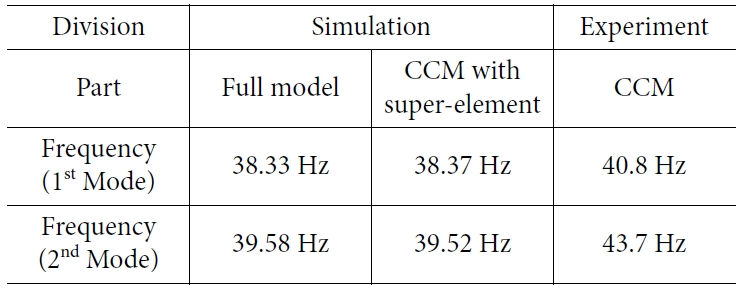conclusion

In this study, we achieved two outcome as follows:
Firstly, the model size of the CCM for the finite element analysis decrease 1/12; in other words, the matrices of model decreased 91% with the same accuracy in terms of full model frequency.
Secondly, sub-models considering the mount of assembly model without assembly model were presented and have the accuracy over 93% compare to experiment. It means if the design of CCM change other type, it is not necessary the full model composed B.I.W. and CCM.
Thirdly, through the hybrid material made by magnesium, aluminum, and engineering plastics, the CCM achieved a reduced weight by 44.9% compared to the conventional CCM.

References

###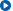Correspondence to

• Daeil Kim
• School of Material Science & Engineering, University of Ulsan

• E-mail: dkim84@ulsan.ac.kr